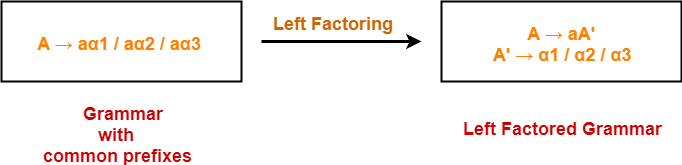# Left Factoring | Left Factoring Examples

## Grammar With Common Prefixes-

 If RHS of more than one production starts with the same symbol, then such a grammar is called as Grammar With Common Prefixes.

### Example-

A αβ1 / αβ2 / αβ3

(Grammar with common prefixes)

• This kind of grammar creates a problematic situation for Top down parsers.
• Top down parsers can not decide which production must be chosen to parse the string in hand.

To remove this confusion, we use left factoring.

## Left Factoring-

 Left factoring is a process by which the grammar with common prefixes is transformed to make it useful for Top down parsers.

### How?

In left factoring,

• We make one production for each common prefixes.
• The common prefix may be a terminal or a non-terminal or a combination of both.
• Rest of the derivation is added by new productions.

The grammar obtained after the process of left factoring is called as Left Factored Grammar.

### Example-Also Read- Left Recursion

## Problem-01:

Do left factoring in the following grammar-

S → iEtS / iEtSeS / a

E → b

## Solution-

The left factored grammar is-

S → iEtSS’ / a

S’ → eS / ∈

E → b

## Problem-02:

Do left factoring in the following grammar-

A → aAB / aBc / aAc

## Solution-

### Step-01:

A → aA’

A’ → AB / Bc / Ac

Again, this is a grammar with common prefixes.

### Step-02:

A → aA’

A’ → AD / Bc

D → B / c

This is a left factored grammar.

## Problem-03:

Do left factoring in the following grammar-

S → bSSaaS / bSSaSb / bSb / a

## Solution-

### Step-01:

S → bSS’ / a

S’ → SaaS / SaSb / b

Again, this is a grammar with common prefixes.

### Step-02:

S → bSS’ / a

S’ → SaA / b

A → aS / Sb

This is a left factored grammar.

## Problem-04:

Do left factoring in the following grammar-

S → aSSbS / aSaSb / abb / b

## Solution-

### Step-01:

S → aS’ / b

S’ → SSbS / SaSb / bb

Again, this is a grammar with common prefixes.

### Step-02:

S → aS’ / b

S’ → SA / bb

A → SbS / aSb

This is a left factored grammar.

## Problem-05:

Do left factoring in the following grammar-

S → a / ab / abc / abcd

## Solution-

### Step-01:

S → aS’

S’ → b / bc / bcd / ∈

Again, this is a grammar with common prefixes.

### Step-02:

S → aS’

S’ → bA / ∈

A → c / cd / ∈

Again, this is a grammar with common prefixes.

### Step-03:

S → aS’

S’ → bA / ∈

A → cB / ∈

B → d / ∈

This is a left factored grammar.

## Problem-06:

Do left factoring in the following grammar-

S → aAd / aB

A → a / ab

B → ccd / ddc

## Solution-

The left factored grammar is-

S → aS’

S’ → Ad / B

A → aA’

A’ → b / ∈

B → ccd / ddc

To gain better understanding about Left Factoring,

Watch this Video Lecture

Next Article- Relationship With Left Recursion

Get more notes and other study material of Compiler Design.

Watch video lectures by visiting our YouTube channel LearnVidFun.

SummaryArticle Name
Left Factoring | Left Factoring Examples
Description
In compiler design, left factoring is a process to transform the grammar with common prefixes. Left Factoring Examples. Problems to perform left factoring on given grammars.
Author
Publisher Name
Gate Vidyalay
Publisher Logo